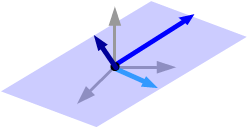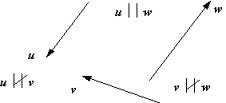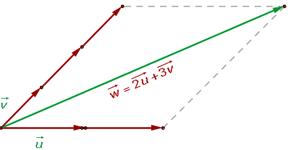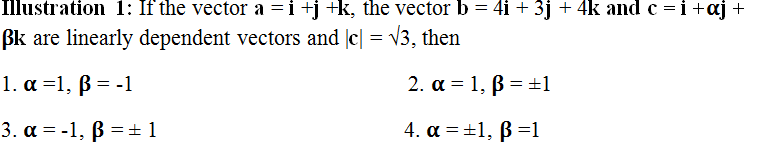×#### Thank you for registering.

One of our academic counsellors will contact you within 1 working day.

Click to Chat

1800-1023-196

+91-120-4616500

CART 0

• 0

MY CART (5)

Use Coupon: CART20 and get 20% off on all online Study Material

ITEM
DETAILS
MRP
DISCOUNT
FINAL PRICE
Total Price: Rs.

There are no items in this cart.
Continue Shopping```Fundamental Theorems of Vectors

Table of Content

Fundamental Theorem of Vectors in Two-Dimensions

Collinear, Coplanar and Parallel Vectors

Fundamental Theorem of Vectors in Three Dimensions

Linear Combination of Vectors

Linearly Dependent and Independent VectorsIn this section, we shall discuss some of the fundamental theorems of Vectors in both two and three dimensions. Here, the letters in boldfaced characters denote the vectors i.e. ‘a’ stands for the vector a.

Fundamental Theorem of Vectors in Two-Dimensions

If a and b be two non-zero non-collinear vectors, then any vector r in the plane of a and b can be expressed uniquely as a linear combination of a and b i.e. there exist unique l, m ∈ R such that la +mb =r.

This also means that if  l1a + m1b = l2a + m2b then l1 = l2 and m1 = m2.

Collinear, Coplanar and Parallel Vectors

Collinear Vectors: The word collinear is used to describe vectors which are scalar multiples of one another. These vectors are parallel and can have different magnitudes in the same or opposite directions.Coplanar Vectors: The word coplanar is used for vectors which are in at least 3 space. As the name suggests, coplanar vectors are three or more vectors which lie in the same plane which could be any two dimensional flat surface.

In other words, the vectors which lie in the same plane or parallel to the same plane are called coplanar. This usually occurs if either the vectors are parallel or they intersect each other. All collinear vectors will also be coplanar. But all coplanar will not be collinear vectors. This point should be remembered.Parallel Vectors: When 2 vectors act along the same line but have a separation between them, they are said to be parallel. i.e. Parallel vectors have the same phase, but different magnitudes. Also, it is due to this only that when two vectors a and b are parallel, a= kb, where k is a constant.

--------A---------> Here A and B are parallel.
----------B--------->Remark: Collinear Vectors are also parallel vectors except that they lie on the same line. When two vectors are parallel, the dot product of the vectors is 1 and their cross product is zero.

Fundamental Theorem of Vectors in Three Dimensions

If a, b and c be three non-zero, non-coplanar vectors in space, then any vector r in space can be expressed uniquely as a linear combination of a, b and c.i.e there exist unique l, m, n ∈ R such that la + mb + nc = r

This also means that if l1a + m1b+ n1c = l2a+ m2b+ n2c, then

l1 = l2, m1 = m2 and n1 = n2.

Linear Combination of Vectors

The linear combination of a finite set of vectors a1,a2,…an is defined as a vector r such that
r = k1a1+ k2a2 + ……+ kn an , where k1, k2, … kn are any scalars (real numbers).Examples

Write the vector m = (1, 2, 3) as a linear combination of the vectors: u = (1, 0, 1), v = (1, 1, 0) and w = (0, 1, 1).

Solution: Let us write the vector m = xu+yv+zw

Then (1,2,3)= x(1,0,1) + y(1,1,0) + z(0,1,1)

(1, 2, 3)= (x+ y, y +z, z +x)

From these we get three equations

x+y=1

y+z=2

x+z=3

Adding these three we get, 2x+2y+2z=6

So x+y+z=3.The first set gives z=2.

The second yield x=1.

From the third we get y=0.

Hence the vector m can be written as a linear combination of vectors u, v and w.

To get an idea about the types of questions asked, you may go through the Papers of Previous Years.

Linearly Dependent and Independent  Vectors

A system of vectors a1,a2,…an is said to be linearly dependent if there exists a system of scalars k1, k2 …, kn (not all zero) such that k1a1+ k2a2 + ……+ kn an= 0

They are said to be linearly independent if every relation of the type

k1a1+ k2a2 + ……+ kn an= 0 implies that k1 = k2 =….= kn = 0.

Notes:

Two collinear vectors are always linearly dependent.

Two non-collinear non-zero vectors are always linearly independent.

Three coplanar vectors are always linearly dependent.

Three non-coplanar non-zero vectors are always linearly independent.

More than 3 vectors are always linearly dependent.

Three points with position vectors a,b,c are collinear if

l1a+l2b+l3c = 0 with l1 +l2+l3 = 0.

Four points with position vectors a,b,c and d are coplanar if

l1a+l2b+l3c+l4d with l1 + l2 + l3 + l4= 0.

Watch this Video for more reference

Illustration 0 : If a,b and c are non–zero non coplanar vectors, determine whether the vectors r1= 2a-3b+c, r2= 3a-5b+2c and r3= 4a-5b+c are linearly independent or dependent.

Key concept:  Three vectors are linearly dependent if they are coplanar that means any one of them can be represented as a linear combination of other two.

Solution: Let r3= xr1+yr2, where x and y are scalars.

If the given vectors are linearly dependent then x and y will exist uniquely; otherwise not.

Consider r3= xr1+yr2

Then 4a-5b+c = x (2a-3b+c) + y(3a-5b+2c)

= (2x+3y)a + (-3x-5y) b + (x+2y)c

But a, b and c are non-zero and non-coplanar vectors.

Hence, 2x+3y = 4    ….(i)

–3x – 5y = –5           …(ii)

x + 2y = 1               …(iii)

Solving (i) and (ii), we get x = 5, y = –2 which also satisfy (iii).

Now x and y are unique so r3= 5r1-2r2.

Hence r1, r2 and r3 are linearly dependent vectors.Solution: Since a, b and c are given to be linearly dependent vectors, so this also implies that the vectors are coplanar.

So, a. (b x c) = 0

Solving this with the help of determinants, and applying the column operation

C3 – C1, we get

(β-1) (3-4) = 0.

This gives β = 1.

Also, |c| = √3

√ 1+ α2 + β2 = √3

√ 1+ α2 +1 = √3

√α2 + 2 = √3

α2 + 2 = 3 so α = ±1.

Illustration 2: If a, b and c are non-planar unit vectors such that

a x (b x c) = (b + c)/√2, then the angle between a and b is

1. 3π/4                                                               2. π/4

3. π/2                                                                  4. Π

Solution: Since a x (b x c) = (b + c)/√2

Hence, (a.c) b – (a.b) c = b/√2 + c/√2

(a.c) = 1/√2 (since b and c are not coplanar)

(a.b) = - 1/√2

cos θ = - 1/√2 (since a and b are unit vectors)

cos 3π/4 = cos θ

θ = 3π/4

Illustration 3: A1, A2, …… , An are the vertices of a regular plane polygon with n sides and O is its center. Show that if the summation runs from 1 to (n-1) then

Σ (OAi x OAi+1) = (1-n) (OA2 x OA1)

Solution: Let v be a unit vector which is perpendicular to the plane of the polygon.

If l is some scalar,

(OAi x OAi+1) = lv, where i = 1, 2, 3, …. , (n-1)

L.H.S = (n-1) lv

Thus, R.H.S = (n-1) OA1 x OA2 (n-1) lv

Hence, L.H.S = R.H.S

To read more, Buy study materials of Vectors comprising study notes, revision notes, video lectures, previous year solved questions etc. Also browse for more study materials on Mathematics here.
```### Course Features

• 731 Video Lectures
• Revision Notes
• Previous Year Papers
• Mind Map
• Study Planner
• NCERT Solutions
• Discussion Forum
• Test paper with Video Solution